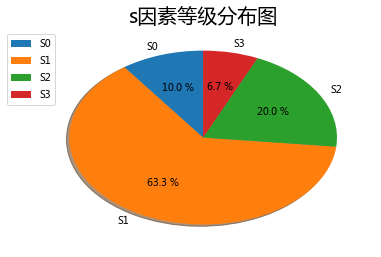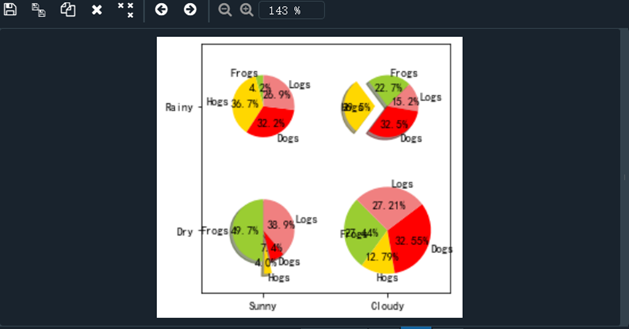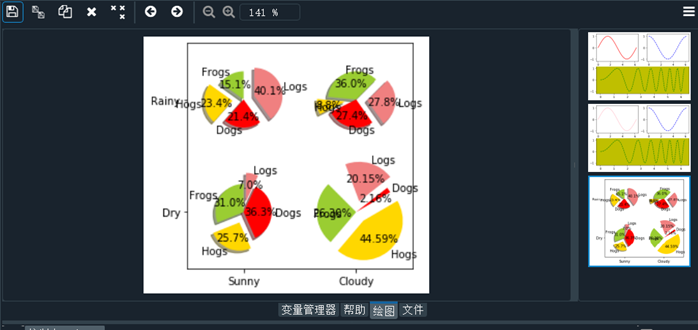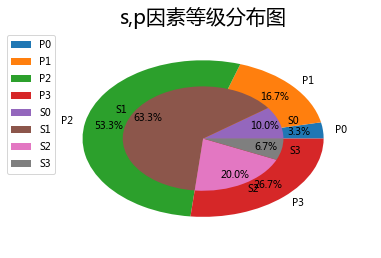• import matplotlib.pyplot as plt plt.rcParams['font.family']= ['SimHei']#设置中文字体...#各种活动在饼状图中的颜色列表 clos = ['c','m','r','b'] plt.pie(slices,labels=activies,colors=clos,shadow=True,exp
import matplotlib.pyplot as plt

plt.rcParams['font.family']= ['SimHei']#设置中文字体

#各种活动标题列表
activies = ['工作','睡','吃','玩']

#各种活动所占时间列表

slices = [8,7,3,6]

#各种活动在饼状图中的颜色列表

clos = ['c','m','r','b']

plt.pie(slices,labels=activies,colors=clos,shadow=True,explode=(0,0.1,0,0),autopct='%.1f%%')

plt.title("绘制饼状图")
plt.show()




展开全文大数据 编程语言
• ## Python绘制饼状图

千次阅读 2017-12-08 10:55:28
已知字典s={'S0': 3, 'S1': 19, 'S2': 6, 'S3': 2}，绘制相应的饼状图代码如下： s={'S0': 3, 'S1': 19, 'S2': 6, 'S3': 2} s_labels=list(sorted(s.keys())) s_fracs=[s.get(s_labels[i]) for i in range...
已知字典s={'S0': 3, 'S1': 19, 'S2': 6, 'S3': 2}，绘制相应的饼状图：
代码如下：
s={'S0': 3, 'S1': 19, 'S2': 6, 'S3': 2}
s_labels=list(sorted(s.keys()))
s_fracs=[s.get(s_labels[i]) for i in range(len(s_labels))]
fig=plt.figure()
plt.pie(x=s_fracs, labels=s_labels,autopct='%3.1f %%',shadow=True, labeldistance=1.1, startangle = 90,pctdistance = 0.6)
plt.legend(loc='upper left', bbox_to_anchor=(-0.1, 1))
plt.title('s因素等级分布图',fontsize=20)
plt.show()
显示结果：展开全文• 本文实例讲述了Python matplotlib绘制饼状图功能。分享给大家供大家参考，具体如下： 一 代码 import numpy as np import matplotlib.pyplot as plt #The slices will be ordered and plotted counter-clockwise. ...
• Python进阶——绘制饼状图 代码需求 绘制饼状图 代码实现 # -*- coding: utf-8 -*- import numpy as np import matplotlib.pyplot as plt labels = 'Frogs', 'Hogs', 'Dogs', 'Logs' colors = ['yellowgreen', '...
Python进阶——绘制饼状图
代码需求
绘制饼状图
代码实现
# -*- coding: utf-8 -*-
import numpy as np
import matplotlib.pyplot as plt

labels = 'Frogs', 'Hogs', 'Dogs', 'Logs'
colors = ['yellowgreen', 'gold', '#FF0000', 'lightcoral'] # 'lawngreen', 'c', 'lightskyblue'

explode1 = (0, 0.1, 0, 0)              #设置突出显示的内容(使饼状图中第2片裂开)
explode2 = (0, 0, 0, 0)

fig = plt.figure()
ax = fig.gca() #当前的子图可以使用plt.gca()获得,然后再调用ax.pie()方法实现真正的绘图。

ax.pie(np.random.random(4), explode=explode1, labels=labels, colors=colors,
autopct='%1.1f%%', shadow=True, startangle=90,
radius=0.25, center=(0, 0), frame=True)   # autopct设置饼内百分比的格式, frame如果是true，绘制带有表的轴框架。
ax.pie(np.random.random(4), explode=explode1, labels=labels, colors=colors,
autopct='%1.1f%%', shadow=True, startangle=45,
radius=0.25, center=(1, 1), frame=True)
ax.pie(np.random.random(4), explode=explode2, labels=labels, colors=colors,
autopct='%1.1f%%', shadow=False, startangle=90,
radius=0.25, center=(0, 1), frame=True)
ax.pie(np.random.random(4), explode=explode2, labels=labels, colors=colors,
autopct='%1.2f%%', shadow=False, startangle=135,
radius=0.35, center=(1, 0), frame=True)

ax.set_xticks([0, 1])                    # 设置坐标轴刻度
ax.set_yticks([0, 1])

ax.set_xticklabels(["Sunny", "Cloudy"])  # 设置坐标轴刻度上的标签
ax.set_yticklabels(["Dry", "Rainy"])

ax.set_xlim((-0.5, 1.5))                 # 设置坐标轴跨度
ax.set_ylim((-0.5, 1.5))

ax.set_aspect('equal')                   # 设置x轴和y轴等长，否则饼图将不是一个正圆

plt.show()

实现结果调整参数后（可自行调整）展开全文数据可视化
• 转自： ... s={'S0': 3, 'S1': 19, 'S2': 6, 'S3': 2} p={'P0': 1, 'P1': 5, 'P2': 16, 'P3': 8} 将上面两个字典绘制在同一张饼状图中： 代码如下： s={'S0': 3, 'S1': 19, '...
转自： https://blog.csdn.net/weixin_40198632/article/details/78749420

s={'S0': 3, 'S1': 19, 'S2': 6, 'S3': 2}

p={'P0': 1, 'P1': 5, 'P2': 16, 'P3': 8}

将上面两个字典绘制在同一张饼状图中：

代码如下：

s={'S0': 3, 'S1': 19, 'S2': 6, 'S3': 2}
s_labels=list(sorted(s.keys()))
s_fracs=[s.get(s_labels[i]) for i in range(len(s_labels))]
p={'P0': 1, 'P1': 5, 'P2': 16, 'P3': 8}
p_labels=list(sorted(p.keys()))
p_fracs=[p.get(p_labels[i]) for i in range(len(p_labels))]
fig=plt.figure()
plt.pie(p_fracs, labels=p_labels,radius=0.9,autopct='%1.1f%%',pctdistance=0.8)
plt.pie(s_fracs, labels=s_labels,radius=0.6,autopct='%1.1f%%',pctdistance=0.8)
plt.legend(loc='upper left', bbox_to_anchor=(-0.1, 1))
plt.title('s,p因素等级分布图',fontsize=20)
plt.show()

显示结果如下：当绘制更多层的饼状图的时候需要更改pie里面的这个radius参数即可
展开全文• 本文实例讲述了Python使用matplotlib的pie函数绘制饼状图功能。分享给大家供大家参考，具体如下： matplotlib具体安装方法可参考前面一篇//www.jb51.net/article/51812.htm，具体使用代码如下： #coding=utf8 ...
• 饼状图绘制 1.利用matplotlib库文件，画出如下的饼图，没有突出显示和图标。 代码显示： import matplotlib.pyplot as plt plt.rcParams['font.sans-serif']=['Youyuan'] labels='A班','B班','C班','D班' sizes=...
• 生成饼状图代码 plt.rcParams['font.sans-serif'] = 'SimHei' # 设置中文显示 plt.figure(figsize=(6, 6)) # 将画布设定为正方形，则绘制的饼图是正圆 explode = [] # 设定各项距离圆心n个半径 Label=[all.index...plt
• 本文实例讲述了Python使用matplotlib的pie函数绘制饼状图功能。分享给大家供大家参考，具体如下：matplotlib具体安装方法可参考前面一篇//www.ddpool.cn/article/51812.htm，具体使用代码如下：#coding=utf8import ...
•   源代码如下： import matplotlib.pyplot as plt plt.rcParams['font.sans-serif']='SimHei'#设置中文显示 plt.figure(figsize=(6,6))#调节图片大小，如果将画布设定为正方形，则绘制的饼图是正圆 label=['第一'...
• 数据可视化之matplotlib绘制饼状图 常常为Python的数据可视化而痴迷，将数据进行可视化只需要掌握相关库的方法使用即可。流水线式的库式调用实现正是显示python强大的库的功能。我们可以绘制各种各样的数据图样式，...数据可视化 可视化
• 前言 通过数据绘图，我们可以将枯燥的数字转换成容易被人们接受的图表，从而让人留下更加深刻的印象。...通过 Matplotlib，可以仅需要几行代码，便可以生成绘图，直方，功率谱，条形，错误，散点，...
• 饼状图是数据可视化中的一种基本图形，常用于展示某一分类变量中各个类别所占的比例。根据饼状图中各个扇形所占的角度大小，可以比较得出数量多的类别和数量少的类别。此外，分类变量可视化的图形还有树形图、玫瑰图...可视化 机器学习 数据可视化 数据分析
• matplotlib是Python的一个强大的2D图形绘制库，使用需要安装奥，通过调用它内部的函数接口，可以清爽的生成直方图、饼状图、功率谱、条形图、错误图、散点图等等 matplotlib安装。 pipinstallmatplotlib 点击...数据可视化 数据分析 可视化
• 我们直接调用matplotlib库中pie()函数来绘制饼状图，pie()函数可以自己计算每个类别所占的比例。代码如下： import matplotlib.pyplot as plt lables=['Nokia','Samsung','Appe','Lumia'] values=[10,30,45,15] ...
• 圆环图本质上是一个中间切出一块区域的饼状图。可以使用python和matplotlib库来实现。本文主要介绍基于matplotlib实现圆环图。本文所有代码见：Python-Study-Notes # 去掉警告 import warnings warnings....
• 目录一、基本图的绘制1.1直线的绘制1.2折线的绘制1.3散点图的绘制1.4绘制不同颜色的散点图1.5绘制不同颜色的线1.6柱状图1.7饼状图1.8直方图1.9三维图1.10等高线图二、样式2.1基本样式2.2画布分区的使用三、函数3.1...数据可视化
• ## python画饼状图

千次阅读 2021-03-05 20:15:16
今天，我们试着用python绘制我们的饼状图 一、引入matplotlib库 二、完整代码 1.如下 代码如下（示例）： import matplotlib as mpl import matplotlib.pyplot as plt mpl.rcParams["font.sans-serif"]=[...matplotlib
• 前言 本文的文字及图片来源于网络,仅供学习、交流使用,不具有任何商业用途...这是饼状图最常见的类型，代码如下： ''' 如有需要Python学习资料的小伙伴可以加群领取：1136201545 ''' #绘制高中同学现在职业占比饼状数据分析
• 数据可视化可以对之前的爬虫项目...对于饼状图、柱形图和散点图的理解基本没有什么问题，但是对于折线图的理解还不是很好，还会去花时间去研究的。 以下是几张图表的实现代码： # 引入相关模块 import matplotlib....数据可视化
• 前序 这次在工作中需要对一个excel表格的数据进行可视化...from pyecharts import Bar,Pie#直方图/饼状图 data = xlrd.open_workbook("xywy.xlsx")#打开文件 table = data.sheets()#取第一个sheet print(table.nrow数据分析 大数据
• 关于动态条形图，小F以前推荐过 Bar、 Chart、 Race 这个库。...最近小 F 又发现一个可视化图库 Pandas_Alive，不仅包含动态条形图，还可以绘制动态曲线图、气泡图、饼状图、地图等。 同样也是几行代码就能完成动态
• Python小白逆袭大神Day3-《青春有你2》选手数据分析作业：请在下方提示位置，补充代码，对《青春有你2》对选手体重分布进行可视化，绘制饼状图数据挖掘 机器学习
• 半小时拿下Python数据处理之Matplotlib篇matplotlib三种代码风格pyplotpylabObject Oriented子图多图散点图（scatter）条形图 (bar)直方图（hist）饼状图（pie）箱型图（boxplot）颜色与样式颜色样式网格（grid）...matplotlib
• 我的Blog 博客案例 记录博客中使用的代码~ PyTorch Tutorials-What is PyTorch：PyTorch安装和...散点图 ，柱状图，饼状图，曲线图，3D图 坐标轴数据，图例属性，注解，多子图 机器学习案例 聚类(kmean) 高斯混合模型
• 读取excel文件数据进行分析绘制条形图和饼状图代码 完整代码如下 联系我们，一起学Python吧 使用python requests库爬取福布斯排行榜数据存放到本地excel文件，并通过matplotlab将数据进行分析和可视化 原网页...可视化 excel
• 文章目录一，绘制单个饼图1，引入库2，显示中文标签3，是否显示负号4，线的粗细5，图例大小6，图大小7，定义饼状图的标签，标签是列表8，每个标签占百分比9，添加图标题10，(1)绘制饼图10，(2)全部代码二，绘制多个...后端# python绘制饼状图代码python 订阅